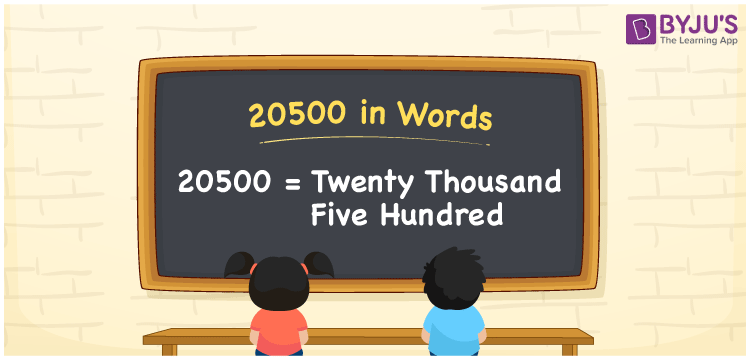# 20500 in Words

20500 in words is written as Twenty thousand five hundred. In both the International System of Numerals and the Indian System of Numerals, 20500 in words is written as Twenty thousand five hundred. The number 20500 is a Cardinal Number as it could represent some quantity. For example, “that laptop costs 20500 rupees”.

 20500 in words Twenty thousand five hundred Twenty thousand five hundred in Number 20500

## 20500 in English Words

20500 in English words is read as “Twenty thousand five hundred.”## How to Write 20500 in Words?

To write 20500 in words, we shall use the place value chart. In the place value chart, put 2 in the ten thousands, 0 in the thousands, 5 in the hundreds and 0 in the tens and the ones, respectively. Let us make a place value chart to write the number 20500 in words.

 Ten Thousands Thousands Hundreds Tens Ones 2 0 5 0 0

Thus, we can write the expanded form as

2 × Ten Thousand + 0 × Thousand + 5 × Hundred + 0 × Ten + 0 × One

= 2 × 10000 + 0 × 1000 + 5 × 100 + 0 × 10 + 0 × 1

= 20000 + 0 + 500 + 0 + 0

= 20500

=Twenty thousand five hundred.

20500 is a natural number, the successor of 20499 and the predecessor of 20501.

20500 in words – Twenty thousand five hundred

• Is 20500 an odd number? – No
• Is 20500 an even number? – Yes
• Is 20500 a perfect square number? – No
• Is 20500 a perfect cube number? – No
• Is 20500 a prime number? – No
• Is 20500 a composite number? – Yes

## Frequently Asked Questions on 20500 in Words

Q1

### How to write 20500 in words?

20500 in words is written as Twenty thousand five hundred.
Q2

### How to write 20500 in the International and Indian System of Numerals?

In both, the system of numerals, 20,500 in words, is written as Twenty thousand five hundred.
Q3

### How to write 20500 in a place value chart?

In the place value chart, write 2 in the ten thousands place, 0 in the thousands, 5 in the hundreds place and again 0 in the tens and the ones place respectively.